# Quadratic Equations

Parent topic:
Equations
•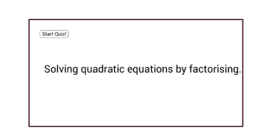### Quadratic Quiz

Activity

Sparky

•### Solving Quadratic Equations Using Quadratic Formula (Quiz)

Activity

Tim Brzezinski

•### Exploring Quadratic Functions

Activity

Sangeeta Gulati

•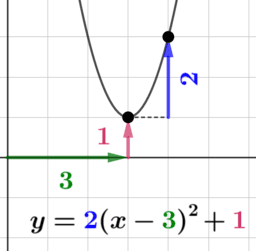### Quadratic Functions Anatomy (3)

Activity

Tim Brzezinski

•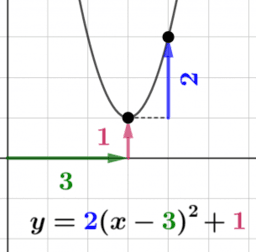### Transforming Quadratic Function Graphs

Book

Tim Brzezinski

•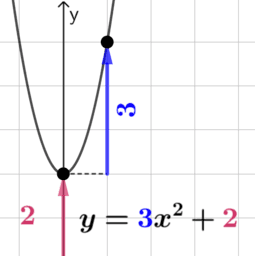### Quadratic Functions Anatomy (2)

Activity

Tim Brzezinski

•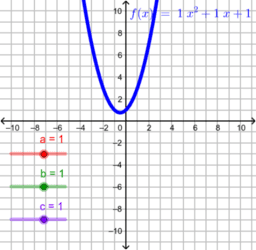### Graphing quadratic equations

Activity

Sabrina Hochman

•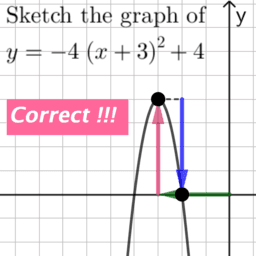### Transforming Graphs of Quadratic Functions (1)

Activity

Tim Brzezinski

•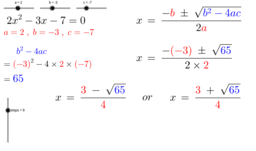### Quadratic Equations - Formula

Activity

David T

•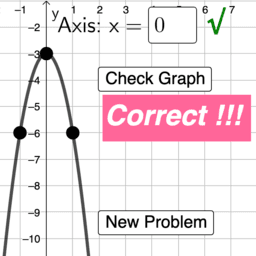### Graphing Quadratic Functions

Activity

Tim Brzezinski

•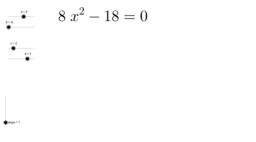### Quadratic Equations-factorising

Activity

David T

•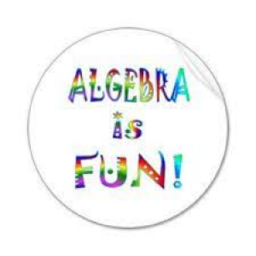### Algebra 1

Book

Mark Beckwith

•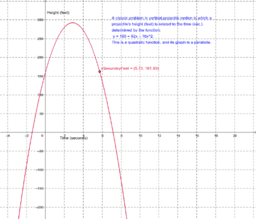### Geom. & Alg.: Quadratics

Book

Terry Lee Lindenmuth

•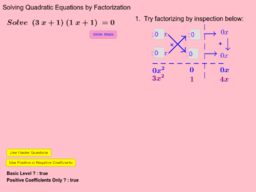### Self Assessment: Solve Quadratic Equations by Factorization

Activity

Lew W. S.

•### Solving Quadratic Equations using Quadratic Formula

Activity

Hisham Amir

•### Quadratic Equations - Super Mario Brothers

Activity

hackingmaths

•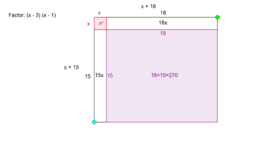### Factoring Area Model

Activity

Steve Phelps

•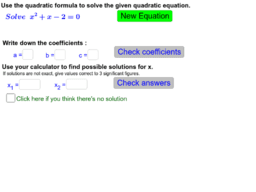### Solving Quadratic Equations using Formula

Activity

Lew W. S.

•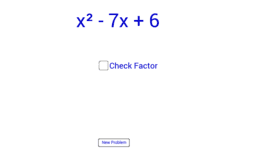### Factoring Quadratics with a Coefficient of One

Activity

smarburger

•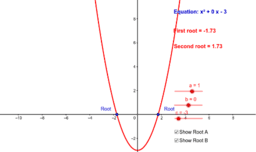### Solving Quadratic Equations

Activity

Folens Publishers

•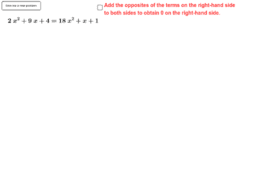### Guided Example: Solving Quadratic Equations using Factorization

Activity

Hisham Amir

•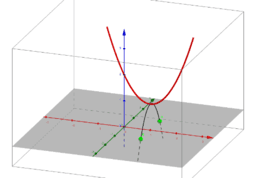### Visualizing Complex Roots of Quadratic Equations

Activity

Aran Glancy

•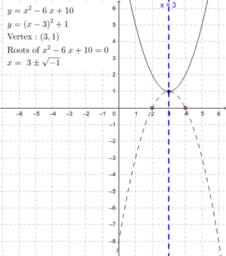### Complex roots of quadratic equations

Activity

GeoGebra Institute of MEI

•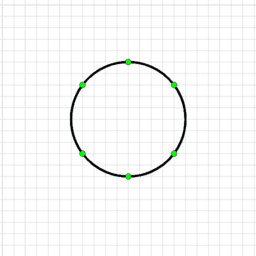Activity

Steve Phelps